## 流程图控件GoJS教程：如何制作影像

# 正版采购狂欢节，企业采购正当时 #

GoJS是一款功能强大，快速且轻量级的流程图控件，可帮助你在JavaScript 和HTML5 Canvas程序中创建流程图，且极大地简化您的JavaScript / Canvas 程序。

GoJS具有用于创建图像的两个功能：Diagram.makeImageData，它输出一个Base64图像数据串，和Diagram.makeImage，这是一个方便的功能调用Diagram.makeImageData并返回一个新HTMLImageElement与图像数据作为其源。这两个函数具有相同的单个参数，即JavaScript对象，其中包含几个可定义的属性，在文档中进行了枚举。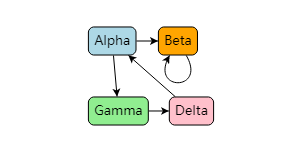`myDiagram.makeImage（）;`

```myDiagram.makeImage({
scale: 1
});```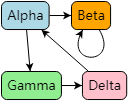```myDiagram.makeImage({
scale: 2
});```

```myDiagram.makeImage({
size: new go.Size(100,100)
});```

```myDiagram.makeImage（{
size：new go.Size（100，NaN）
}）;```

``` myPartsList = new go.List();
myDiagram.makeImage({
parts: myPartsList
});```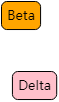```myDiagram.makeImage（{
}）;```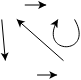```myDiagram.makeImage({
scale: 1.5,
size: new go.Size(100,100)
});```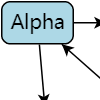maxSize的默认值为go.Size(2000, 2000)，并且指定go.Size(600, NaN)等效于指定go.Size(600, 2000)。如果我们不想在高度上裁剪，我们可以写go.Size(600, Infinity)。
```myDiagram.makeImage（{
scale：9，
maxSize：new go.Size（600，NaN）
}）;```

```myDiagram.makeImage({
position: new go.Point(20,20),
size: new go.Size(50,50)
});```

```myDiagram.makeImage（{
size：new go.Size（NaN，250），
background：“ rgba（  0，255，0，0.5 ）” //半透明的绿色背景
}）;```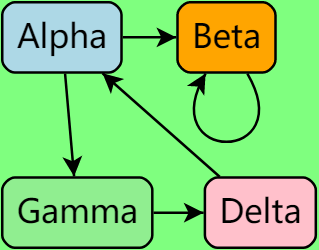```var d = myDiagram.documentBounds;
var halfWidth = d.width / 2;
var halfHeight = d.height / 2;
img = myDiagram.makeImage({
position: new go.Point(d.x, d.y),
size: new go.Size(halfWidth,halfHeight)
});

img = myDiagram.makeImage({
position: new go.Point(d.x + halfWidth, d.y),
size: new go.Size(halfWidth,halfHeight)
});

img = myDiagram.makeImage({
position: new go.Point(d.x, d.y+ halfHeight),
size: new go.Size(halfWidth,halfHeight)
});

img = myDiagram.makeImage({
position: new go.Point(d.x + halfWidth, d.y + halfHeight),
size: new go.Size(halfWidth,halfHeight)
});```myDiagram.makeImage（{
scale：1，
type：“ image / jpeg”
}）;```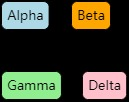```myDiagram.makeImage({
scale: 1,
background: "AntiqueWhite",
type: "image/jpeg"
});```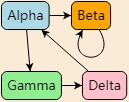```myDiagram.makeImage({
scale: 1,
background: "AntiqueWhite",
type: "image/jpeg",
details: 0.05
});```

### 为你推荐

• 推荐视频
• 推荐活动
• 推荐产品
• 推荐文章
• 慧都慧问相关厂商相关产品
GoJS

GoJS是一款功能强大，快速且轻量级的流程图控件，可帮助你在JavaScript 和HTML5 Canvas程序中创建流程图。热门标签 全部相关文章 MORE